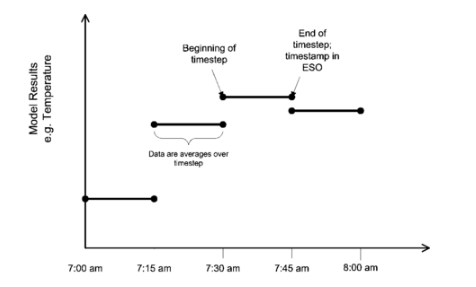Question-and-Answer Resource for the Building Energy Modeling Community
Get started with the Help page

# Documentation for relation of output to timestepI have been looking around quite a while, but did not find a particular documentation.

In EnergyPlus what is the output (e.g. mean zone air temperature or humidity ratio or relative humidity) at 01.01.2002 06:00:00?

1. the current value at 6:00:00
2. the average value from 5:01:00 to 6:00:00

3. the average value from 6:00:00 to 6:59:00

4. the average value from 5:30:00 to 6:30:00

I would assume it is option 2 according to the weather file format definition. But I don't know.

But is there a documentation on it?

edit retag close merge delete

Sort by » oldest newest most voted

### 1. What does the timestep represent?

According this diagram from the timestep model formulation documentation, the timestamp represents the end of the timestep.### 2. What length of time does the value represent?

This depends on value of the Reporting Frequency field of the Output:Variable or Output:Meter used to generate the output. According to the documentation, there are several options:

Field: Reporting Frequency

This field specifies how often the variable will be listed in the output file. “Detailed” will list the value each calculation step (i.e. Zone or HVAC). “Timestep” will be the same as “Detailed” for Zone valued variables and will be aggregated to the Zone timestep (i.e. Timestep in Hour value) for HVAC variables. “Hourly” will aggregate the value to the hour. “Daily” will aggregate to the day (i.e. one value per day). “Monthly” will aggregate to the month (i.e. one value per month). “RunPeriod” will aggregate to the runperiod specified (each Design Day is a runperiod as is each runperiod object). Default for this field if left blank or omitted is Hourly.

### 3. What does the value represent (average, sum, etc.)?

Generally, there are two ways to figure this out:

The first way is to look at the documentation for the object's outputs. So for Zone Mean Air Temperature, we can see that the value is an average.

Zone Mean Air Temperature [C]

From the code definition, the zone mean air temperature is the average temperature of the air temperatures at the system timestep. Remember that the zone heat balance represents a “well stirred” model for a zone, therefore there is only one mean air temperature to represent the air temperature for the zone.

The second way is to look in the .rdd file that is generated after you run a simulation. This file lists all of the Output:Variables available for this model. You will notice that although Zone Mean Air Temperature is an average at the specified frequency, some of the other variables are a sum across the specified frequency. A sum really makes sense when you are looking at how much something (energy, water, etc.) was used during that period.

Output:Variable,*,Water Heater Mains Water Volume,hourly; !- HVAC Sum [m3]
Output:Variable,*,Zone Mean Air Temperature,hourly; !- Zone Average [C]
Output:Variable,*,Zone Operative Temperature,hourly; !- Zone Average [C]

more